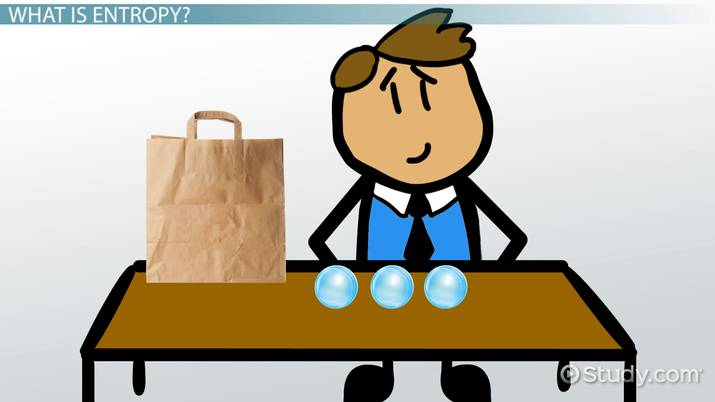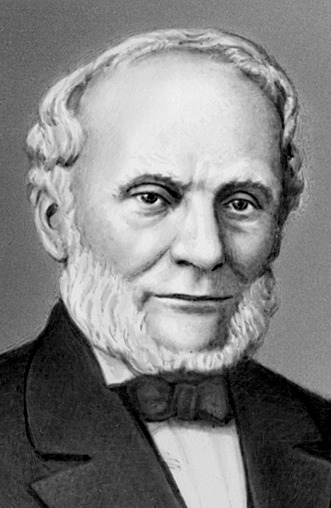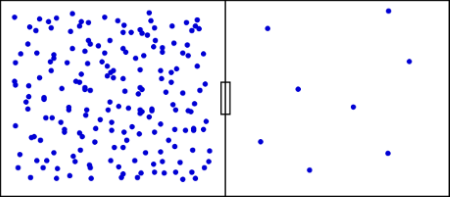# Law of Entropy

Mary Gutierrez, Richard Cardenas, Elaine Chan
• Author
Mary Gutierrez

Mary has her Masters in Sustainable Community Development, a certification in Public Health with an Environmental Health focus, and B.S. in Environmental Science all from the University of West Florida. She has over 20 years as an Environmental Professional and has several years of curriculum development and teaching Environmental Science, Biology, and Marine Science.

• Instructor
Richard Cardenas

Richard Cardenas has taught Physics for 15 years. He has a Ph.D. in Physics with a focus on Biological Physics.

• Expert Contributor
Elaine Chan

Dr. Chan has taught computer and college level physics, chemistry, and math for over eight years. Dr. Chan has a Ph.D. in Chemistry from U. C. Berkeley, an M.S. Physics plus 19 graduate Applied Math credits from UW, and an A.B. with honors from U.C .Berkeley in Physics.

What is entropy? Learn the definition and formula of entropy. Also learn about entropy specific to gases, examples that use the entropy formula, and applications. Updated: 08/28/2021

Show

## Entropy Definition

Entropy is the measure of how disorganized and random a system is. It is related to the Second Law of Thermodynamics. The Second Law of Thermodynamics states that unless outside energy is provided, a system will find its entropy (disorder) staying the same or increasing as time goes on. In other words, a system will never get more ordered without outside intervention.An error occurred trying to load this video.

Try refreshing the page, or contact customer support.

Coming up next: What Is Geothermal Energy? - Definition, Advantages & Disadvantages

### You're on a roll. Keep up the good work!

Replay
Your next lesson will play in 10 seconds
• 0:00 What Is Entropy?
• 1:09 The Second Law of…
• 2:18 Change in Entropy
• 3:00 Multiplicity
• 4:19 Lesson Summary
Save Save

Want to watch this again later?

Timeline
Autoplay
Autoplay
Speed Speed

## Development of Entropy Concept

The concept of entropy was developed in the early 1850s by Rudolf Clausius due to a response to an observation that a certain amount of functional energy released from combustion reactions is lost to dissipation or friction, thereby not transferring the energy into useful work. Clausius put forth the concept of the thermodynamic system and argued that, in an irreversible process, a small amount of heat energy is incrementally dissipated across the system boundary. He continued to develop the ideas of lost energy and coined the term entropy.## Entropy Equation

In equations, entropy is usually denoted by the letter S and has units of joules per kelvin {eq}\left ( J \cdot K^{-1} \right ) {/eq} or {eq}kg \cdot m^{2} \cdot s^{^{-2}} \cdot K^{-1} {/eq}. A highly ordered system has low entropy.

During entropy, the process is defined as the amount of heat emitted or absorbed isothermally and reversibly divided by the absolute temperature. If we add the same quantity of heat at a higher temperature and lower temperature, randomness will be maximum at a lower temperature. This suggests that the temperature is inversely proportional to the entropy.

### Entropy Examples

A campfire is an example of entropy. The solid wood burns and becomes ash, smoke, and gases, all of which spread energy outward more easily than the solid fuel.

Here are two examples that use the entropy formula to find a solution.

Combustion Reaction: {eq}C_{2}H_{8}(g))+ 5O_{2}(g) \rightarrow 3CO_{2}(g)+ 4H_{2}O(g) {/eq}

{eq}\Delta H = -2045kJ {/eq}

The reaction is exothermic so there is an increase in the surrounding entropy. An exothermic reaction is indicated by a positive

{eq}\Delta S {/eq} value. This means heat was released to the surrounding area or that the environment gained energy. This is a positive change in entropy. Although the step by step reactions are not shown here, the change in entropy of the surroundings of reaction is {eq}\Delta Ssurr {/eq}= 6.86 kJ/K or 6860 J/K.

Endothermic Reaction: {eq}H_{2}O(l)\rightarrow H_{2}O(g) {/eq}

{eq}\Delta H = ^{+}44kJ {/eq}

This reaction needed energy from the surroundings to proceed and reduced the entropy of the surroundings. A negative ?S value indicates an endothermic reaction occurred, which absorbed heat from the surroundings. Although the step by step reactions are not shown here, the change in entropy of the surroundings is {eq}\Delta Ssurr {/eq} = -0.15 kJ/K or -150 J/K## Applications of Entropy

Almost every chemical and biological process is governed by changes in entropy (and free energy). Here is a real-world example that should help you understand this concept.

In bioenergetics, many of the reactions that take place in living organisms require a source of free energy to drive them. The immediate source of this energy in heterotrophic organisms, which include animals, fungi, and most bacteria, is the sugar glucose. Oxidation of glucose to carbon dioxide and water is accompanied by a large negative free energy change. It can be shown in the following equation:

{eq}C_{6}H_{12}C_{6} + 6O_{2}\rightarrow 6CO_{2}+ 6H_{2}O \Delta G^{^{0}} = -2,880 kJmol^{^{-1}} {/eq}

Of course, it would not do to simply burn the glucose in the normal way; the energy change would be wasted as heat, and rather too quickly for the well-being of the organism! Effective utilization of this free energy requires a means of capturing it from the glucose and then releasing it in small amounts when and where it is needed. This is accomplished by breaking down the glucose in a series of a dozen or more steps in which the energy liberated in each stage is captured by an energy carrier molecule, of which the most important is adenosine diphosphate, known as ADP. At each step in the breakdown of glucose, an ADP molecule reacts with inorganic phosphate and changes into ATP:

Where does the glucose come from? Animals obtain their glucose from their food, especially cellulose and starches that, like glucose, have the empirical formula ({eq}CH_{2}O {/eq}}. Animals obtain this food by eating plants or other animals. Ultimately, all food comes from plants, most of which are able to make their own glucose from {eq}CO_{2} {/eq}and {eq}H_{2}O {/eq} through the process of photosynthesis.

### The First Law of Thermodynamics

The First Law of Thermodynamics states that energy can never be generated or destroyed but can only change into different forms. The First Law of Thermodynamics relates to the principles of entropy because, within a system, the entropy of one system can decrease by raising the entropy of another system. Therefore, the entropy of the system is neither generated nor destroyed, but changed into a different form.

### The Multiplicity Image

Where kB is the Boltzmann constant (also written as k) and is equal to 1.38065 * {eq}10^{-23} {/eq} J/K, it shows the relationship between entropy and the number of ways the atoms or molecules of a thermodynamic system can be arranged. It links the entropy of a system (macroscopic quantity) to the number of equilibrium microscopic states that the system allows. The system is a constant (number of particles (N), Volume (V), total energy (E), or constant (N, V, T). The Boltzmann equation links the macroscopic worlds.

The number of microstates corresponding to a given macrostate is called the multiplicity g(E,V). Multiplicity becomes a fundamentally important quantity since the macrostate with the highest multiplicity is the most probable macrostate.

To unlock this lesson you must be a Study.com Member.

## Calculate Entropy

#### Problem:

50 kg of water at 20 ° C is mixed with 50 kg water at 24 ° C. Estimate the change in entropy without using calculus.

Since we start with equal amounts of water, the final temperature will be 22 ° C. As the hot water cools down from 24 ° C to 22 ° C, an amount of heat equal to Q= mc Δ T = (50 kg ) (1 kcal / kg K) ( 2 ° C) = 100 kcal flows out of the hot water. Heat Q, flows into the cool water as it heats up from 20 ° C to 22 ° C . The total change in entropy is the sum of the change in entropy of the hot water plus the change in entropy of the cold water. We estimate entropy change as Δ S = Q / Tav , where Tav is 23 ° C ( 296 ° K ) for the hot water. The average temperature for the cold water is 21 ° C ( 294 ° K ) . The change in entropy of the hot one is - (100/ 296) kcal / ° K = - 0.338 kcal / ° K. The heat flows out of the hot system, giving the entropy a negative sign. The change in entropy of the cold system is ( 100/ 294 ) kcal/ ° K = 0.340 kcal / ° K . The total change in entropy is ( -0.338 + 0.340 ) (kcal / ° K) = 0.002 kcal / ° K. The total change in entropy is positive.

### Register to view this lesson

Are you a student or a teacher?